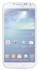#### MobileMathr

Collection of formulas out of mathematics and physics. The special feature is that the application can calculate the...

#### Love-math

This fun apps will reinforce basic arithmetic concepts and spark a love for learning math. Don't let your kids waste their time playing on the phone. Have them practicing math instead.In this game you will calculate math examples so quickly as you can. Try to answer the questions correctly and increase the scale of the...

#### Random Math Table

Random Math Table by Girish Padia V 2.00 S60 V5 S^3 Anna Belle J2ME This application is useful the kids who just learn the mathematics table 1 to 10 and 11 to 20. This will help them to practice on it. don't require data...

#### AnyUok Science Manual

Science Manual is combination of Unit Convert, Scientific Constants and Chemical Elements. It contains more than 676 units grouped in 38 categories, 112 chemical elements and more than 110 scientific constants sorted in 5...

#### Ruler

This app turn your Phone into a handy pocket ruler that can measure in both inches and centimeters. Features: - Elegant design - Measure in inches and...

#### operation matrix

Always had trouble with those difficult matrices sums? here's help-operation matrix! that performs matrix operations for...

#### Mobile Maths v1.7

1. Professional Calculator 2. Equation Solver 3. Definite Integration 4. Function Solver 5. Linear System Solver 6. Powerful Graph 2D 7. Powerful Graph 3D 8. Powerful Curve Fitting (Regression) 9. Statistics 10. Matrix Operations 11. Interpolation using Cubic Spline 12. Unit Conversion 13. Powerful Periodic Table 14. Powerful Molecular weight calculations 15. Opening files from phone/card...

#### MobileMaths v1.7

Thereas no doubt that Mobilemath is a greatest math apps for symbian s60v5 edition. This math apps really awesome because it has so many function such as equation, Integral, function, Periodic Table, Statistics, Matrics function, Powerful Curve Fitting Regression, Interpolation using...